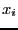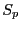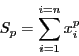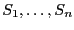Next: Viète relations Up: Bounds on the product Previous: Mathematical background   Contents

Implementation

Letbe theroots (either complex or real) of a polynomial of degree. Letbe:This procedure enable to compute theelements. The syntax is:

VECTOR SumN_Polynomial_Interval(int Degree,VECTOR &Coeff)
with:
• Degree: degree of the polynomial
• Coeff: coefficients of the polynomial ordered along increasing degree
This procedure returns 0 if the leading coefficient is equal to 0, 1 otherwise. There is an equivalent procedure for interval polynomial:

INTERVAL_VECTOR SumN_Polynomial_Interval(int Degree,INTERVAL_VECTOR &Coeff)
which returns intervals including the. This procedure returns 0 if 0 is included in the leading interval.

Jean-Pierre Merlet 2012-12-20